• GeoXD
• GTS NX
Overview Geometry Modeler Mesh Generator Solver System Post-Processing
• Events
• Resources
Blog MIDAS Expert Network Technical Support
• Get Started
GeoXD GTS NX
• Talk to usIntroduction

Rock mass modeling is one of the most important challenges for the design of rock tunnels, as it has to deal with relevant discontinuity systems and complex anisotropic behaviors. This document presents some calculation methods to simulate the mechanical behavior of anisotropic rocks: continuum models (FEM - finite element method and FDM - finite difference method) and discontinuum models (DEM - discrete element method). An example of calibration of the input parameters and analysis of the excavation of a deep tunnel in an anisotropic formation is dealt with using three three-dimensional modeling software: continuum with the use of Midas GTS NX and of another FEM software, discontinuum with the use of 3DEC. A comparison of the numerical models developed is presented, with a highlight on the different capabilities of the methods and the importance of input parameter calibration to simulate the rock mass behavior.

# Rock Mass Modeling

The compressive strength of the rock mass is dependent on the properties of the rock blocks and joints, as well as the angle formed by the direction of the compressive stress and its projection onto the plane of weakness. The discrete elements numerical method is considered as the most suitable for modeling rock mass with an important presence of discontinuities, as it explicitly simulates the presence of micro or macro discontinuities, in accordance with the scale of the analyzed problem.

To analyze the behavior of the rock mass in a simplified way, equivalent continuum models are frequently used, in which the behavior of the layered material can be simulated by an equivalent homogeneous anisotropic medium; alternatively, it is possible to consider constitutive models that simulate the presence of discontinuities, or else to model the rock mass as a continuum medium in which interfaces are explicitly modelled to simulate the presence of the main discontinuities. These latter simplified models are referred to as pseudo-discontinuum models.

Following the approach proposed by Kassa and Nordal (2016), a calibration study of the input parameters for the pseudo-discontinuum models was conducted, in order to simulate the behavior observed in discrete element models. The parameters considered in the calibration study were the mechanical properties of the intact rock blocks and of the main discontinuities of the rock mass.

This study consisted in the simulation of uniaxial compression laboratory tests (UCS) on samples of stratified sedimentary rocks with a layer spacing of about 1m. The obtained results with different numerical calculation methodologies were compared, in terms of uniaxial compressive strength and stiffness. Ten samples were studied, differing in the angle of inclination of the discontinuities with respect to the horizontal axis, varying from 0 ° to 90 ° with a spacing of 1m.

The three types of numerical models used are summarized in Table 1 and are detailed in the following paragraphs. Figure 1 presents an example of the numerical analysis carried out for the calibration of the input parameters of the discontinuities. These figures show the deformations generated in the different planes of discontinuity in the simulation of the UCS test, clearly highlighting the failure planes of the rock mass.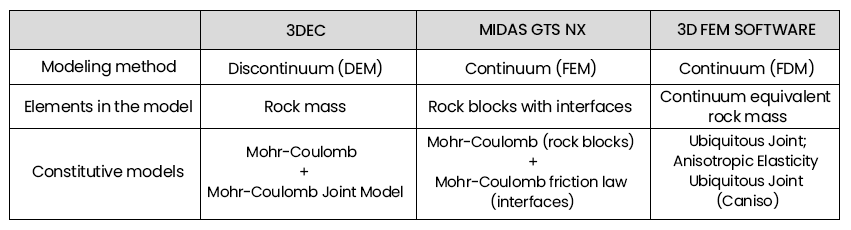Table 1. Numerical analysis methods used for the modeling of rock mass.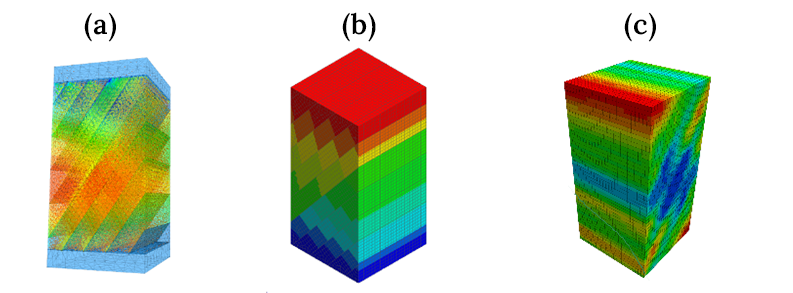Figure 1. Numerical simulation of uniaxial compression laboratory tests: representation of the deformations generated along the failure planes on the samples of the rock mass in a) 3DEC; b) MIDAS GTS NX; c) 3D FEM software.

## 1) Discontinuum model: discrete elements method (DEM) in 3DE

Initially, the simulations of the uniaxial compression tests in 3DEC were carried out, evaluating the input parameters of the main discontinuities by comparing the results obtained with the geotechnical laboratory investigations performed on sedimentary rock samples. In 3DEC the rock mass samples are modeled through the Discrete Frame Network (DFN), which considers the geometric distribution of the main discontinuities within the rock mass. The constitutive model considered for the rock blocks is the Mohr-Coulomb elasto-plastic model and the Mohr-Coulomb Joint Model for discontinuities. The comparison between the results obtained from the numerical simulation and the values ​​of the laboratory tests allowed to estimate the correct mechanical input parameters for the software.

## 2) Continuum model: finite element method (FEM) in Midas GTS NX

The rock mass samples were modeled as blocks of rock, made with 3D elements, divided by interface elements to simulate the presence of the main system of discontinuities. For the rock blocks a Mohr-Coulomb equivalent elasto-plastic constitutive model was used, deriving the input parameters from the Hoek-Brown model, while the Mohr-Coulomb friction law was used for the interfaces.

The tests simulated in Midas GTS NX follow the behavior observed in the discrete element analyses in both the elastic and plastic fields.

## 3) Continuum model: finite difference method (FDM) in another 3D FEM software

The Ubiquitous Joint constitutive model was used, which simulates the presence of the main discontinuities in rock mass by characterizing the material with a Mohr-Coulomb failure envelope and at the same time considering the shear strength of the discontinuities as a tension limit (cut -off).

The Anisotropic Elasticity Ubiquitous Joint constitutive model was also used in the test simulations, which exclusively considers the anisotropic behavior in terms of stiffness of the rock mass.

After analyzing the results obtained from the simulations, it is concluded that these constitutive models prove to be simplified approaches, as they do not represent the anisotropy of the behavior of the rock mass in both the elastic and plastic fields. The Ubiquitous Joint constitutive model represents the anisotropy of the rock mass only in the plastic field, while the Anisotropic Elasticity Ubiquitous Joint constitutive model simulates the anisotropic behavior only in the elastic field.

## 4) Presentation of the calibration results

The rock mass uniaxial compressive strength can be analytically calculated using the approach proposed by Jaeger & Cook (1979).

Figure 2 shows the obtained uniaxial compressive strength for the different rock samples: the above-described models are able to simulate the failure behavior of the samples both when it is governed by the strength of the rock, i.e. for very low or very high inclinations of the discontinuities, and when the failure occurs along the discontinuity planes of the rock mass, i.e. for intermediate angles of the discontinuities.

Midas GTS NX and the 3D FEM software with Ubiquitous Joint Model

# Case Study

The case study consists of a 16m height and 15m width “D shape” tunnel, at a depth of 360m below ground level. The site presents sedimentary rocks with predominantly sub-horizontal discontinuities at 1m spacing, for which a support system of 4m long rock bolts every 1.5m and spritz beton lining has been provided.

## 1) Discontinuum model: 3DEC

The Discrete Frame Network (DFN) of the rock mass was defined, considering the presence of the main discontinuity systems and attributing the mechanical properties as illustrated in the previous section. The excavation sequence was simulated modeling the rock bolts as axial structural elements.

Figure 3 shows the calculation model (considering three systems of discontinuity for the rock mass domain) and the vertical displacements obtained after the tunnel excavation and the rock bolts installation. The radial convergences, the displacements of the single rock blocks, the plasticization of the rock mass around the excavation profile and the axial forces generated in the rock bolts were analyzed.

## 2) "Pseudo-discontinuum" model: Midas GTS NX

The explicit modeling technique of discontinuities by means of interface elements was adopted as illustrated in the previous section. This way of modeling was performed in a geometric domain around the tunnel, without extending the interfaces to the entire modeled rock mass domain after having verified that the extension of the rock mass yield zone was well within this geometric domain, as shown in Figure 4: in this way excessive computational costs were avoided. The rock bolts were modeled as axial structural elements and the spritz beton lining with shell elements, ensuring the node connection between the bolts and the lining.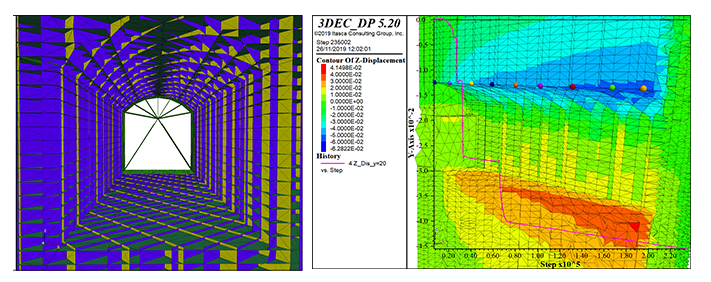Figure 3 Modeling of the tunnel and vertical displacements (in meters) of the crown during excavation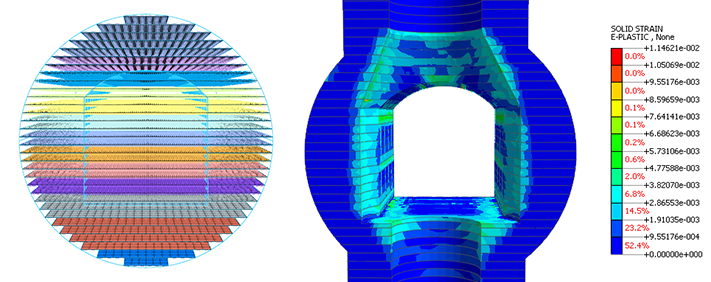Figure 4 Modeling in Midas GTS NX of the discontinuities as interface elements and verification of the yield zone

# Conclusion

Discontinuum modeling with DEM provides a rigorous analysis of the deformation and yield processes of anisotropic rock mass. However, the generation of the DFN in 3DEC and the calibration of the mechanical parameters of the main and secondary discontinuities are difficult to determine and the numerical models require enormous computing costs. Some constitutive models to simulate the anisotropy of rocks such as the Ubiquitous Joint Model and the Anisotropic Elasticity Ubiquitous Joint are simplified and have limitations, failing to simulate the anisotropic behavior in both the elastic and plastic fields. Continuum-based analysis methods, where discontinuities are explicitly simulated through interfaces, allow to improve and simplify modeling with some limitations, especially with respect to the number of discontinuity systems that can be represented. For the case study, the results obtained with the two different calculation methods 3DEC and Midas GTS NX are comparable. The choice of the analysis methodology strongly depends on the geological and geotechnical characterization of the rock mass, the geometric scale of the problem to be analyzed, the details of the required calculations and the numerical analysis tools available.

# Bibliography

1. Kassa, S. Nordal (2016). Numerical models on anisotropy of rocks. NGM 2016-17th Nordic Geotechnical Meeting.
2. C. Jaeger & N. G. W. Cook (1979). Fundamentals of Rock Mechanics. 3rd edition. Chapman and Hall. London, UK.
3. Itasca Consulting Group (2017). 3DEC - 3-Dimensional Distinct Element Code - Manual V 5.2. Minneapolis, USA.
4. MIDAS (2019). GTS NX User Manual
5. J.S. Bolivar Vasco, F. Brambilla (2021). Analisi numerica al continuo e al discreto di gallerie in formazioni anisotrope. X IAGIG, Pisa, Italy.

##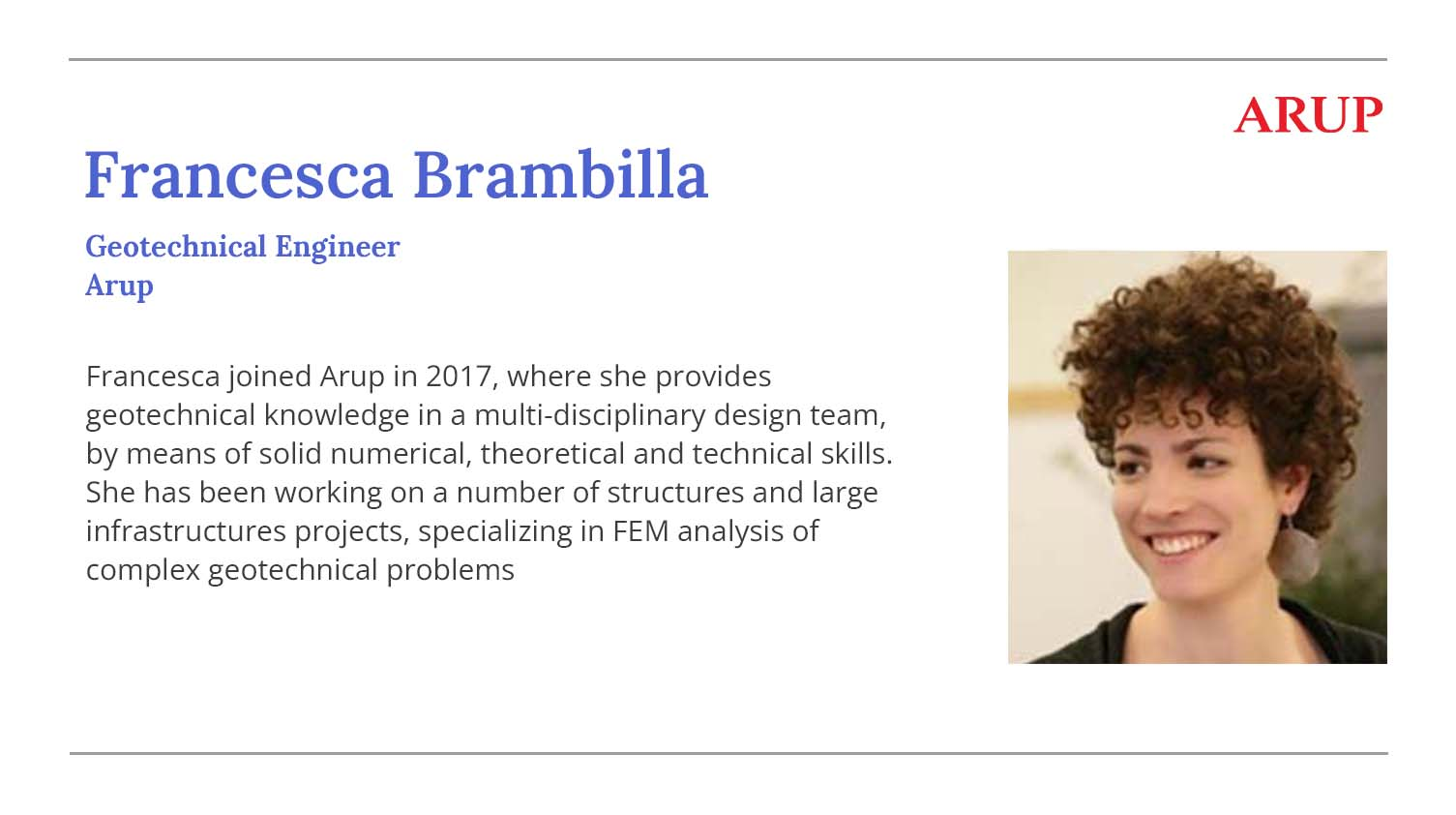👉 Relevant project

▪️ Project Stories | Interface between new railway tunnel and subway extension tunnel

Topics midas GTS NX#### Written by Hyebin Cha

Want Engineering tips